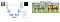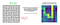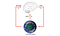# A Brief Introduction for Reinforcement Learning

This post is based on the material I prepare for a learning session in my department. I have also made a slide and recorded video, but I cannot share them for corporate reasons.

Compared with some classic machine learning tasks, reinforcement learning (RL) is not easy to learn. It’s very easy to get lost among all those concepts without a system of knowledge. Here I will guide you through my knowledge system for reinforcement learning and hopefully get you started in this interesting field.

This post is organized as follows.

1. First, I will introduce what RL does with an example.
2. Then, I will explain some fundamental concepts.
3. Next, after we have learned those concepts, we will look at two main categories of RL algorithms, where most RL algorithms will fall into one or both.
4. Finally, we will finalize by a quick note about some common problems in RL.

Note that I am still a learner and by no means an expert on reinforcement learning. Therefore, this post, which merely represents my humble perspective, is surely lack of depth and not even accurate enough. If you find any problems, please point them out :-)

# What RL Does

To answer this question, let’s look at another question here. Suppose we want to train a machine learning model that solves the maze like below

• Input: Maze Matrix
• Output: Solution Path

If you are familiar with computer vision, you might tempt to use a popular technique called image to image translation, where we input a maze image, and get a maze image with path drawn as the output. Can we solve the maze problem with it?Image To Image Translation Demonstration. Left: Typical neural architecture . Right: Example 

Probably, No

• A simple observation is that pixel distance does not translate to solution closeness, makes the maze difficult to solve with just one forward inference.
• However, confidently, we believe it is possible to solve this problem with a series of decisions.

Reinforcement Learning (RL) is about solving problems that need series of decisions.How RL may Solve a Maze (I draw this heatmap by hand, so it does not have an exact correspondence to the maze on the left, but you get the ideas.)

With RL, we need to design a reward function, e.g. have +100 on the exit, -100 on the dead-end. Given that reward, an RL agent will learn the expected future reward on each position, which describes the goodness of the position. Then we just follow the warm area of the heatmap, we can reach the exit.

Applications of RL are two-faceted. On the one hand, we are witnessing some amazing cases like better scheduling cluster computation , neural architecture search , simulated robot control , gaming , and etc. However, on the other hand, companies like DeepMind have been losing money for years.

Personally, I would conclude that the applications of RL are still in their early stages. Here I quote some words from “Yes, Minister”: Comparing to football, art is what people don’t want at this moment, but ought to have.

RL is likely in a similar position.

# Fundamental Concepts

Next, we will introduce some fundamental concepts of Reinforcement Learning.

## Observation, Actions and Rewards

As we said before, RL is about solving problems that need series of decisions. Therefore, we need to model the decision process. Here we model it as a sequence of observations, actions, rewards:

Then, we will have the first and probably the most fundamental hypothesis of RL, the reward hypothesis.

Reward Hypothesis: All goals can be described by the maximization of expected cumulative reward.

It assumes that the goal of an RL agent is to maximize its expected cumulative reward, or we can say the sum of all rewards we can collect along the decision process. It is very interesting to think about this hypothesis.

Suppose an ascetic agent only cares about rewards in the end, he will likely be an exception from the above hypothesis.The agent observes the environment, and reacts to its observation with an action, then gets a reward from the action taken. Full Observability means Oₜ = Sₜ, we usually use Sₜ.

## Markov Hypothesis and Policy

After we learn the reward hypothesis, let us look at the second one, the markov hypothesis. It assumes that the current state only depends on the previous state, and can greatly simplify the formulation.

This assumption greatly simplifies the problem formulation and stimulates many beautiful mathematical findings, but it also restricts us from more possibilities like distributive state representation.

With the help of this markov hypothesis,

• We can represent the environment with a transition probability P(Sₜ₊₁ | Sₜ, Aₜ)
• We only need to act based on current state, i.e. we can represent our policy with π(Aₜ|Sₜ)

## Reward, Return and Value Function ⭑

We almost finish with fundamental concepts here, but this section is a very important one.

Recall the reward hypothesis, RL agent optimizes towards expected cumulative reward. Therefore, we need to first define Reward Rₜ

Reward: Rₜ is a real value random variable, depends on the value of Sₜ, Aₜ.

Then we look at the cumulative reward. Ideally, we want to calculate cumulative rewards by summing all the rewards from the beginning state to our goals. But we don’t know how to get to the goals or even what goals are there; therefore, we approximate it by looking forward from the current state Sₜ. We define the Return Gₜ as follows:

Keep in mind that Gₜis still a random variable.

Finally, we define the expected cumulative reward, by taking the expectation of Gₜ on π and P, namely the probability of policy and environment. We call them value function V and Q, they are the targets we need to optimize.

• The V function measures the usefulness of a state s
• The Q function measures the usefulness of applying action a at a state s

By expanding the expectation, we can easily see that Q and V can represent each other. I would like to highlight that the concepts in this section are frequently used, especially for the value function. It is worth spending more time on it.

To demonstrate these concepts, let’s take a look at an example of super mario:

# Two Main Categories of RL algorithms

After we have learned these fundamental concepts, let’s look at what algorithms that we can build on top of these concepts. Here, I will introduce two categories of RL algorithms, and most RL algorithms can fall into one or both of these categories.

1. Bellman Equation based Iterative Optimization

Let’s take a look at them in the following.

## Bellman Equation based Iterative Optimization

Let’s take a look at the first category. To learn about the bellman equation, we need to introduce another concept called optimal policy.

Optimal Policy

Surely there are good policies and bad policies. Since we optimize towards the expected cumulative reward, a good policy will have generally higher value function and vice versa.

And here we have a theorem, for any Markov Decision Process (MDP)

1. There exists an optimal policy π* ≥ π, ∀ π
2. Optimal policy achieves optimal value function V* and Q*
3. If we have Q*(s, a), then π*(a’ ∣ s) = 𝕀(a’ = argmaxₐ Q*(s, a))

Basically, it tells us that for any decision process like we model before, if we have the optimal Q* function, then we can recreate the optimal policy π*. Therefore, we can say that Q* and π* are equivalent.

So that being said, if we can represent the Q function with a neural network, given the neural network is a universal function approximator, and if we can train the neural network well, then we will have a good policy.

Optimal Substructure

But how do we train the network? Well, it turns out, the value function has an optimal substructure, which is a typical pattern we find for dynamic programming. The substructure exists because of the definition of Gₜ.

These two equations are called Bellman Expectation and Optimality Equation. The first one just follows from the definition of Q​ and V​; while the second one, recall the equivalency of ​Q* and V*​, is also straight-forward.

We can particularly leverage the optimality equation. since it is true for the optimal value function, we can just force our neural network to behave this way.

Iterative Optimization

This is actually what the Deep Q-Network (DQN)  does, where the loss function comes directly from the optimality equation.

So this is the first main category of RL algorithms. There are actually numerous RL algorithms that are designed upon the bellman equation. We won’t cover them, but you can get the idea from this example.

Here I provide additional details for this section.

(1) Derivation of Bellman Expectation Equation:

(2) Why we can converge by optimize iteratively:

Let us define

We can see that

Therefore, by applying the contraction operator iteratively, the Q function will converge into the Q*.

(3) Since it is usually hard to compute P in practice, we will usually apply some tweaks to make the P implicit, like in Q-learning.

Now, let’s look at the second category. Suppose we can represent the policy π(a|s) with a neural network, the idea is to directly optimize the policy network, rather than optimize Q function and then derive a good policy.

The goal here is still to maximize the expected cumulative reward, namely the value function. But how do we calculate the gradient of the policy?

Here I will just provide you the final answer, we can calculate the gradient of the policy network following the equation below, which we called the policy gradient theorem.

Then, to train a model with the policy gradient, we can

• Approximate the expectation with mini-batch sampling (common deep learning practice)
• Represent πθ(a|s) with a neural network
• Approximate with a discounted sum of samples of rewards rₜ + γ rₜ₊₁ + … by definition.

Until now, we have learned the two main categories of RL algorithms.

Proof of Policy Gradient Theorem (Optional)

The proof of policy gradient theorem is elegant and is a good reading practice to understand those concepts. I summarize the proof in the following.

Actor Critic (Optional)

High Variance: Cumulative reward depends on consecutive steps, which means a small change in ​π will bring large shift in gradient, especially when the policy is still being baked.

• Approximating with samples of reward is unbiased, but will introduce high variance. We can reduce variance by introducing a small bias.
• We can replace with Qw, i.e. another neural network (aka Critic). And the policy network πθ is usually called Actor.
• Qw can be trained simultaneously using bellman equation approach in the last section, while πθ is trained using policy gradient.

## Typical RL Algorithm implementation looks like

After we learning the two categories of RL algorithms, let us look at how an algorithm is usually implemented. An RL algorithm can contain these steps:

1. Collecting trajectories by playing the current agent in the environment
2. Sample batches of sₜ, aₜ, sₜ₊₁, rₜ, and update the parameters of the agent
3. Collect trajectories again using the updated agent

# Final Notes

We have been talking about the benefits of reinforcement learning, but here let’s take a short break to watch an example of RL failure.

RL usually needs a massively amount of cheap samples, which is not very realistic to real-world robots, and delicate reward function design, while a flawed reward function will result in strange behavior like above.

Therefore, we say applications of RL are still in the early stages and mostly focus on domains that have relatively complete digital models at this moment.

Reinforcement Learning is a broad area, there are also lots of topics we can talk about, like

• Need of Exploration, and its trade-off to Exploitation
• Trade-off between Variance and Bias that can be seen in lots of algorithms
• How to do planning (e.g. Monte Carlo Tree Search ), if you have a model of the environment

To ensure that this post is not too long, I will not cover these topics. To learn more, a good starting point is always the marvelous course by David Silver .

This post will finish here. I hope it is useful to you. Thank you for your reading and see you then 😀

# Reference

: U-Net: Convolutional Networks for Biomedical Image Segmentation https://arxiv.org/abs/1505.04597

: Unpaired Image-to-Image Translation using Cycle-Consistent Adversarial Networks https://arxiv.org/abs/1703.10593

: Device Placement Optimization with Reinforcement Learning https://arxiv.org/abs/1706.04972

: Neural Architecture Search with Reinforcement Learning https://arxiv.org/abs/1611.01578

: Trust Region Policy Optimization https://arxiv.org/abs/1502.05477

: Mastering Chess and Shogi by Self-Play with a General Reinforcement Learning Algorithm http://arxiv.org/abs/1712.01815

: PyBullet, a Python module for physics simulation for games, robotics and machine learning http://pybullet.org/

: Stable Baseline3 https://github.com/DLR-RM/stable-baselines3

: MarI/O — Machine Learning for Video Games https://www.youtube.com/watch?v=qv6UVOQ0F44

: Playing Atari with Deep Reinforcement Learning https://arxiv.org/abs/1312.5602

: Kernel-Based Least Squares Policy Iteration for Reinforcement Learning https://ieeexplore.ieee.org/document/4267723

: Policy Gradients in a Nutshell. Everything you need to know to get https://towardsdatascience.com/policy-gradients-in-a-nutshell-8b72f9743c5d

: Learn AI Game Playing Algorithm Part II — Monte Carlo Tree Search https://xyzml.medium.com/learn-ai-game-playing-algorithm-part-ii-monte-carlo-tree-search-2113896d6072

: Introduction to Reinforcement Learning with David Silver https://deepmind.com/learning-resources/-introduction-reinforcement-learning-david-silver

SDE @ Microsoft